# Simulation Environment

This page describes the details of developing a robust and accurate simulation environment. An accurate simulation combines the equations of motion derived in the System Modeling section, the specific parameter values estimated in the Model Verification section, and the controller designed in the Controller Design section. All of these pieces are necessary to create an accurate simulation environment. An accurate simulation environment enables operators to design and test control designs, filters, observers, and path planning algorithms before they are implemented on the physical system in the real world. The following sections outline the code necessary to implement a quadrotor simulator in MATLAB. Where appropriate, specific code or blocks are highlighted in greater detail.

The Matlab scripts developed allow a user to quickly and easily make small modifications such as physical parameters or control gains as well as large changes such as the equations of dynamics or controller types. It also allows the user to quickly generate data and manipulate the plotting of the data for analysis purposes. Below is a brief description of the main files and functions required to run and manipulate the simulated quadrotor.

The simulator is run using the quadrotor_sim.m file. This file initializes the simulation environment with the following commands. The global variable Quad is created which will hold all of the quadrotor variables.

``` %% Add Paths

%% Initialize Workspace
clear all;
close all;
clc;

%% Initialize the plot
init_plot;

%% Initialize Variables

The function init_plot.m draws the three dimensional environment which the quadrotor’s simulated movement will be visualized inside of. Next, plot_quad_model.m is called. This script begins by loading the Quad_plotting_model.mat file which is created by running define_quad_model.m. This .mat file uses the quadrotor’s physical dimensions such as arm length, arm thickness, and propeller radius to define the vertices of each arm and motor in three dimensions for plotting purposes. The plot_quad_model.m script then draws the initial quadrotor model in the three dimensional plotting environment.

In the next section, quad_variables.m is run which defines the primary variables for the simulation including the quadrotor’s physical parameters, initial and desired conditions, simulation parameters, and controller gains. The values of the physical parameters are taken from the Model Verification section. The quad_dynamics_nonlinear.m function uses the initial values to calculate the initial velocities and accelerations of the quadrotor model. These simulation uses the nonlinear equations of motion derived in the System Modeling section. The code representing these equations is below. Note that discrete integration is used to calculate the velocities and positions from the acceleration values.

```function quad_dynamics_nonlinear

%% Update Accelerations

The next section in quadrotor_sim.m is a loop which runs the actual simulation once all the initialization is complete. The relevant code is shown below.

``` %% Run The Simulation Loop

%% Measure Parameters (for simulating sensor errors)
sensor_meas;

%% Filter Measurements (future work)

%% Implement Controller
&nbsp;&nbsp;&nbsp; position_PID;
&nbsp;&nbsp;&nbsp; attitude_PID;
&nbsp;&nbsp;&nbsp; rate_PID;

%% Calculate Desired Motor Speeds

%% Update Position With The Equations of Motion

%% Plot the Quadrotor's Position
drawnow
end

Quad.init = 1;  %Ends initialization after first simulation iteration

end```

The loop runs for the length of time specified by t_plot. The first two areas are reserved for implementing functions to simulate sensor noise as well as implementing a filter such as an EKF to filter the sensor measurements before sending them to the controller. Currently, only the sensor noise simulation script is implemented. This is done in the sensor_meas.m file. This file updates the global position, linear acceleration, and rotational rate variables using the sensor model derived in the Model Verification section. The specific function is detailed below.

```function sensor_meas

%% GPS Measurements

%% Barometer Measurements

end

%% IMU Measurements

Next, the position_PID.m function is called which acts as the position controller. The outputs of this function, a desired roll and pitch angle, are inputs to attitude_PID.m which is the attitude/altitude controller. Both are PID implementations and implement the equations derived in the Controller Design section. The translational PID controller code is shown below for reference. Note that the desired positions in the global frame must be converted to the body coordinate frame.

```function outer_PID

%% Rotate Desired Position from GF to BF (Z axis rotation only)

%% Rotate Current Position from GF to BF

%% Rotate Current Velocity from GF to BF

%% X Position PID controller
x_error_sum = x_error_sum + x_error;
end
cp = Quad.X_KP*x_error;    %Proportional term
ci = min(Quad.theta_max, max(-Quad.theta_max, ci));    %Saturate ci
Quad.theta_des =  - (cp + ci + cd);   %Theta and X inversely related

%% Y Position PID controller
y_error_sum = y_error_sum + y_error;
end
cp = Quad.Y_KP*y_error;    %Proportional term
ci = min(Quad.phi_max, max(-Quad.phi_max, ci));    %Saturate ci
Quad.phi_des = cp + ci + cd;

end```

The attitude/altitude controller code is shown below. The outputs of this controller are the three desired angular rates and the desired thrust.

```function attitude_PID

%% Z Position PID Controller/Altitude Controller
z_error_sum = z_error_sum + z_error;
end
cp = Quad.Z_KP*z_error;         %Proportional term
ci = min(Quad.U1_max, max(Quad.U1_min, ci));    %Saturate ci
Quad.U1 = -(cp + ci + cd)/(cos(theta)*cos(phi)) + (Quad.m * Quad.g)/(cos(theta)*cos(phi));   %Negative since Thurst and Z inversely related

%% Attitude Controller
%% Roll PID Controller
phi_error = Quad.phi_des - phi;
phi_error_sum = phi_error_sum + phi_error;
end
Quad.p_des = cp + ci + cd;

%% Pitch PID Controller
theta_error = Quad.theta_des - theta;
theta_error_sum = theta_error_sum + theta_error;
end
Quad.q_des = cp + ci + cd;

%% Yaw PID Controller
psi_error = Quad.psi_des - psi;
psi_error_sum = psi_error_sum + psi_error;
end
Quad.r_des = cp + ci + cd;

end```

Lastly, the rate controller is implemented in the rate_PID.m function. This control loop takes the desired angular rates computed from the attitude controller and calculates the remaining three control inputs. These are then mapped to the motor commands in subsequent code.

```function rate_PID

%% Angular Rate Controller
%% Roll PID Controller
p_error = Quad.p_des - p;
p_error_sum = p_error_sum + p_error;
end
Quad.U2 = cp + ci + cd;

%% Pitch PID Controller
q_error = Quad.q_des - q;
q_error_sum = q_error_sum + q_error;
end
Quad.U3 = cp + ci + cd;

%% Yaw PID Controller
r_error = Quad.r_des - r;
r_error_sum = r_error_sum + r_error;
end
Quad.U4 = cp + ci + cd;

end```

Next, the function quad_motor_speed.m is used to convert the control inputs into motor speeds, saturate the motor speeds based on the motor characteristics, and then recompute the control inputs for the equations of motion. The relevant code computes the control input to motor speed mapping formulas derived in the Controller Design section.

```function quad_motor_speed

%% Calculate motor speeds (rad/s)^2

%% Apply realistic motor speed limits
if w1 > Quad.max_motor_speed^2
end
if w1 < Quad.min_motor_speed^2
end

if w2 > Quad.max_motor_speed^2
end
if w2 < Quad.min_motor_speed^2
end

if w3 > Quad.max_motor_speed^2
end
if w3 < Quad.min_motor_speed^2
end

if w4 > Quad.max_motor_speed^2
end
if w4 < Quad.min_motor_speed^2
end

Quad.O1 = sqrt(w1);    % Front M
Quad.O2 = sqrt(w2);    % Right M
Quad.O3 = sqrt(w3);    % Rear M
Quad.O4 = sqrt(w4);    % Left M

%% Re-compute traditional control inputs

end```

After computing the control inputs using the physical motor limits, the control inputs are input into the quadrotor dynamics function, quad_dynamics_nonlinear.m, to update the quadrotor’s position and attitude.

Last in the simulation loop, the plot_quad.m function is called every three iterations to plot the current position of the quad in the three dimensional environment. This allows the user to visualize the behavior of the quadrotor. This function updates the vertices of the quadrotor arms and motors using the position and attitude of the quadrotor output from the equations of motion. An example of this visualization is shown below.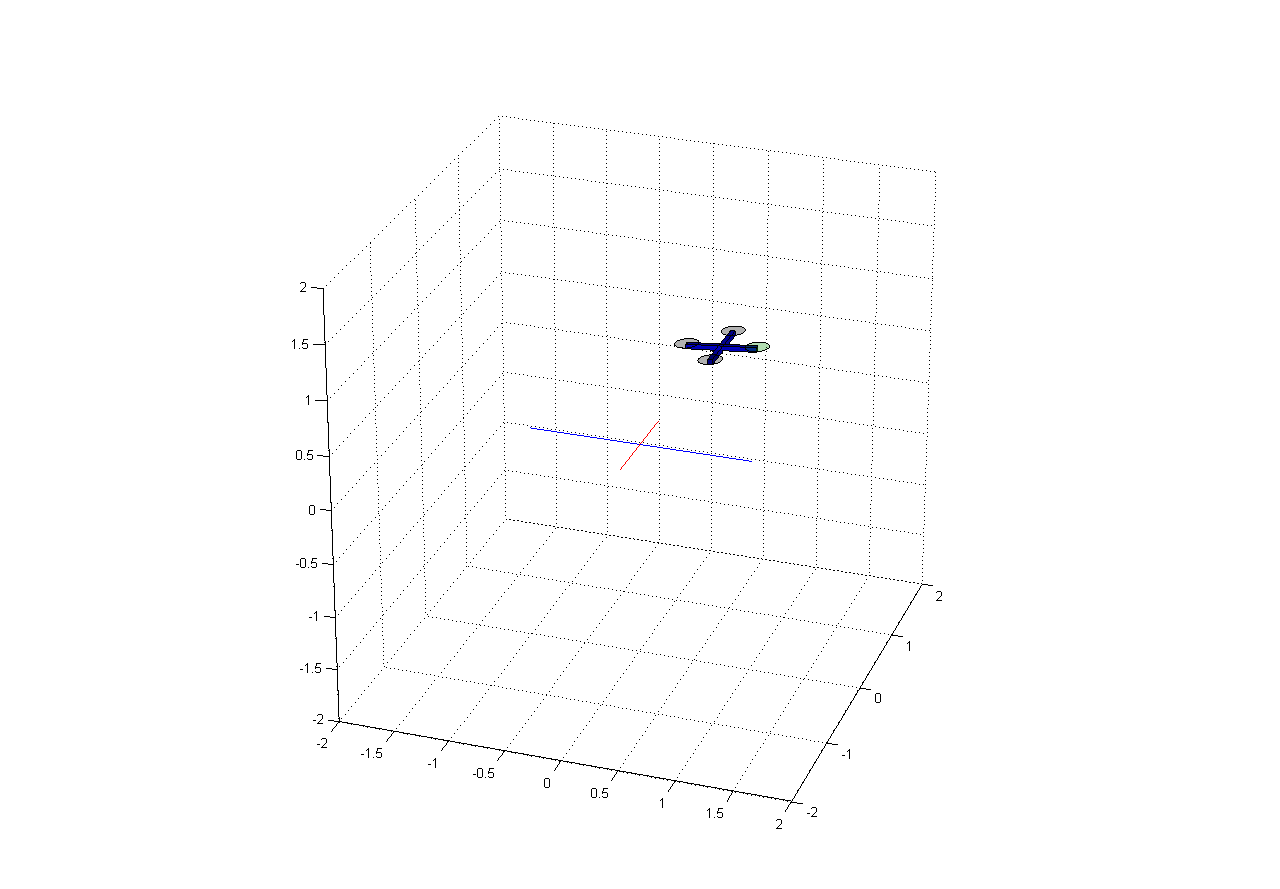Once the simulation is completed, the function plot_data.m is called. This function is used to plot various performance metrics of the quadrotor over the time of the simulation. Plots include the actual and desired positions and attitude angels as well as the calculated control inputs. These plots can be used to manually tune the PID controller gains to specific performance characteristics. A sample plot depicting the system’s response to step inputs is shown below validating the control system design and simulation.An alternative to using the quadrotor simulation script is the use of a Simulink block diagram. The block diagram is stored in the QuadrotorSimulink.mdl file. This file consists of several layers which will be described in detail. There are a couple of benefits with this method. Since the code is stored in blocks, it is easy to switch between different controller or quadrotor dynamics. Simulink also supports blocks such as step inputs and scopes which can be used to analyze and tune the controller parameters. While the variables and parameters can be loaded from a script file, it is also possible to use the block diagram to identify and estimate unknown system parameters using real world flight data. An overview of the simulation is shown below. The step function values and scopes can be used to manipulate the quadrotor initial conditions and analyze the system’s simulated behavior. The simulation can be run from the QuadSimulink.m file which also plots some of the data.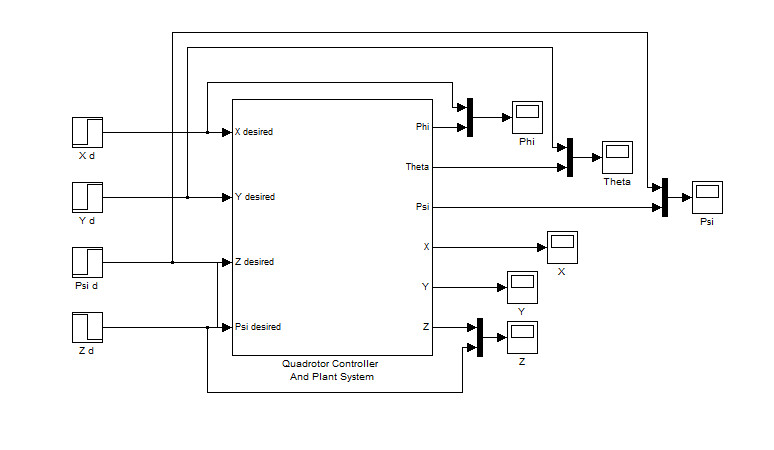At the top level, the block diagram is broken down into 3 primary blocks. These include the outer loop position controller, the inner loop attitude/altitude controller, and the quadrotor dynamics. This series of blocks as well as their inputs and outputs it shown below.Stepping into the translational position controller reveals the following block diagram. Note that these equations do not depict equations to compute a desired yaw. Typically, the user sends the autopilot yaw commands that translate into a desired yaw rate. This way, the pilot can manipulate the yaw and have the quadrotor maintain a constant heading when the yaw stick is centered. If the yaw commands translated to a specific angle, the quadrotor would rotate back to its original heading when the yaw stick was centered. These equations calculate a desired yaw rate based on a desired yaw angle input. However, the desired yaw rate is typically taken directly from the RC command inputs.The desired roll and pitch angles computed by the position controller are combined with the desired altitude and heading in the attitude/altitude controller shown below.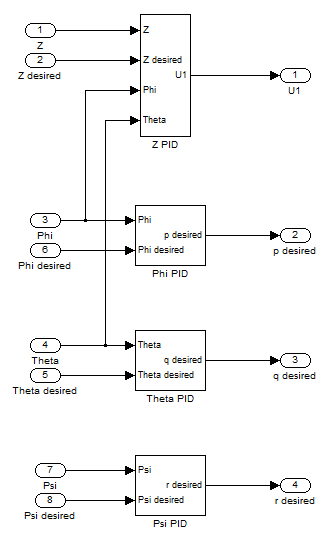The altitude controller computes the desired thrust control input from the desired altitude. The attitude controllers take the desired angles and compute desired angular velocities for the final rate controllers.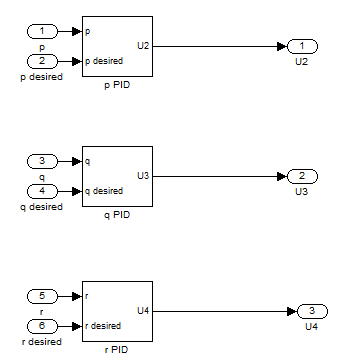The angular velocity controller computes the final three moment control inputs. Each PID block contains the individual Proportional, Integrator, and Derivative gains shown in the generic block diagram below and detailed further in the Control Systems Design section.The final inputs are then fed into the quadrotor dynamics block. As shown below, this system is made up of several smaller subsystems.The motor speed calculator limits the control inputs to the physical motor parameters.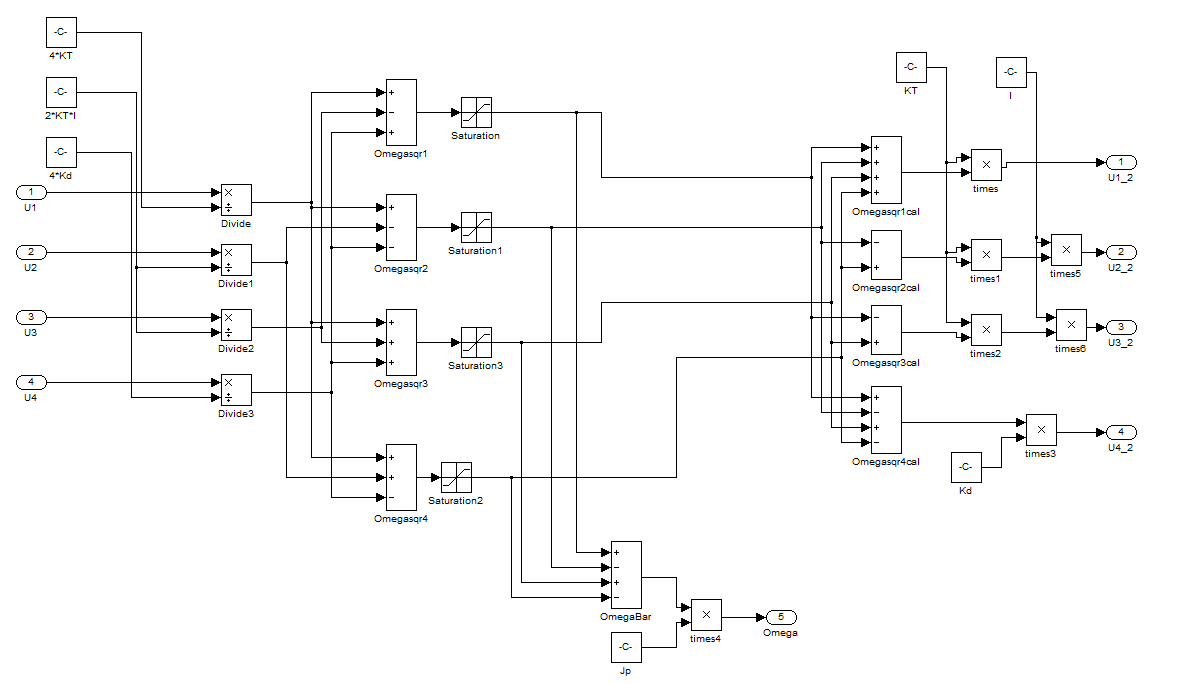The rotational dynamics calculate the angular accelerations.These values are fed into the angular velocities transformation block which computes the angular velocities.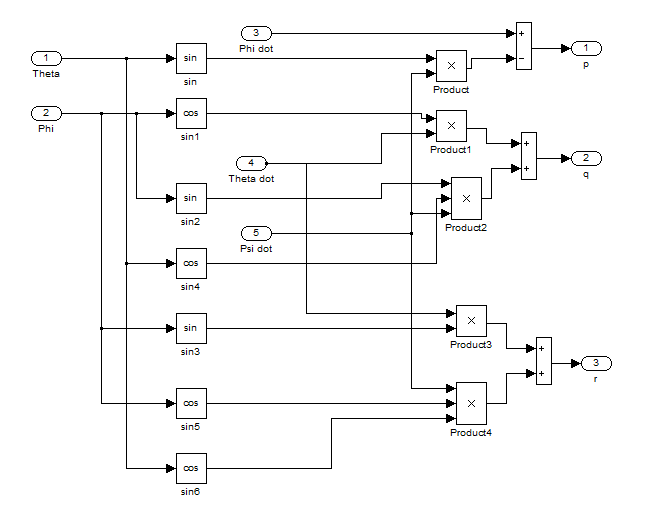The angles are also fed into the translational dynamics block which computes the translational accelerations.A sample plot depicting the system’s response to step inputs is shown below validating the control system design and simulation.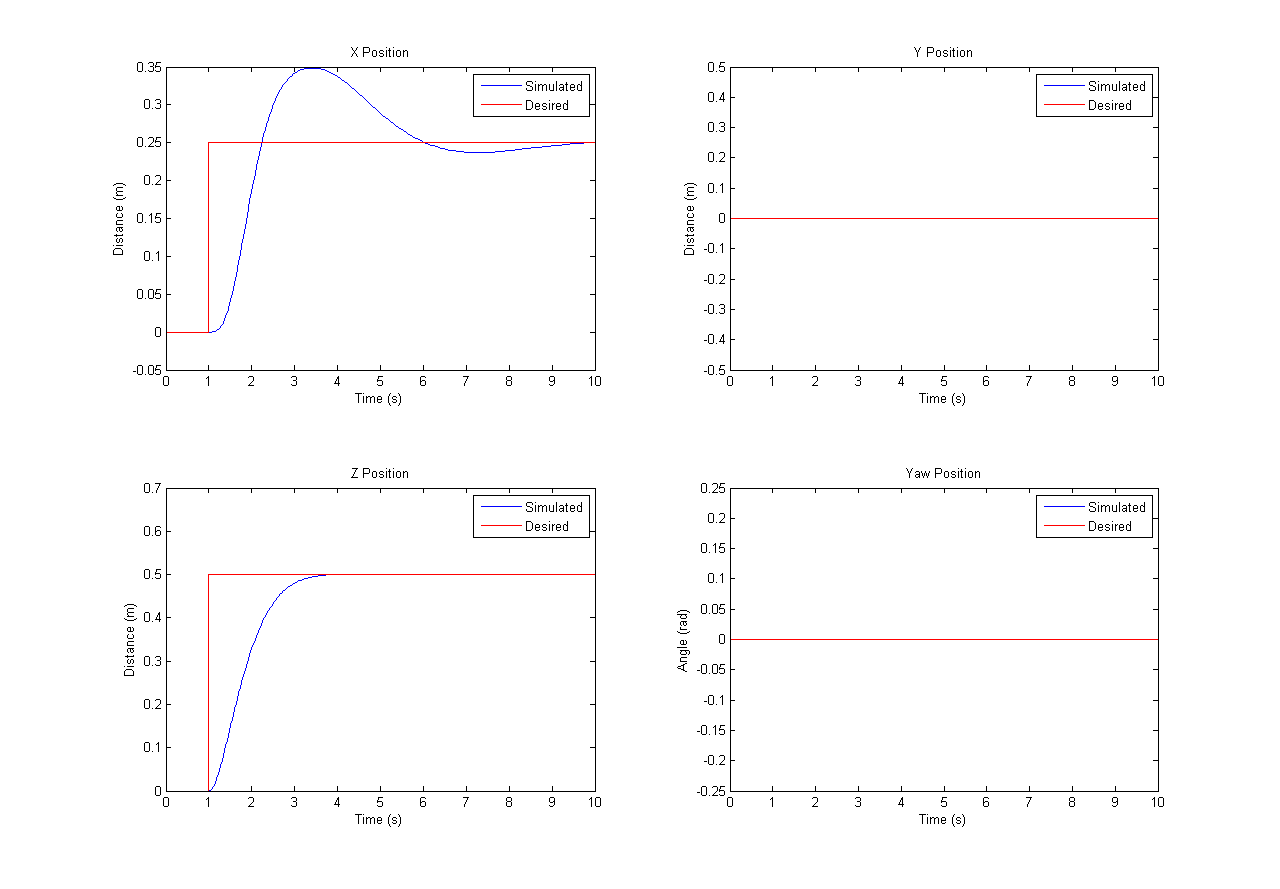## 29 thoughts on “Simulation Environment”

1.Akmal Sabri says:

Hi Sir. I am student from Malaysia. I interested with your work especially this project. I already look at your code. but can I know where to tune the PID gain in utilities. Thanks !!

1.wilselby says:

Check out quad_variables.m. You can edit everything there. Good luck!

2.Ronello Ninettu says:

Hello! I’m researching on PID of a quadrotor, and your work is really amazing! However, is there any way to vary the desired roll and pitch?… because I’ve tried varying the x and y desired values however I always get an error…. I’m pertaining to the Simulink model.. since I cant seem to change the desired values in the code version

Thanks! and amazing work!

1.wilselby says:

Ronello,

For the script file, the desired values are stored in quad_variables.m as Quad.X_des_GF and Quad.Y_des_GF = 1. In the Simulink model, you should be able to update the desired position by editing the Xd and Yd step function block as inputs to the Translational Position PID controller.

1.Ronello Ninettu says:

Hello, Mr. Selby! First of all, thank you for your quick response! May I ask another question?

I’m currently working on the PID control of the ArduCopter code… and I was wondering where the mass/weight of the quadrator was considered… if it was considered at all… Even just the parameter name is helpful enough…And may I ask if the paramter AP_MOTORS_THST_HOVER_DEFAULT is related somehow to the weight/mass?

Thank you!

1.wilselby says:

Ronello,

I’m not very familiar with the full ArduCopter code but I think you are on the right track. At a stable hover, the thrust value must equal the downward force of gravity acting on the quadrotor, which includes it’s mass. AP_MOTORS_THST_HOVER_DEFAULT is the estimates throttle at hover so that takes into account the weight implicitly. To maneuver, deviations from this hover thrust are calculated and sent to each motor. The resulting change in attitude results in a transnational motion.

If you wanted to include mass in your calculations, you could map throttle values to total force of all the motors combined. You could then equate this to the downward force of gravity (m*g). If the mass of the quadrotor changes, then the estimated hover thrust value would change as well. This might not be done in the code since it’s fairly simple to pilot the quadrotor manually and identify the average thrust value. It doesn’t need to be perfect since there are controllers on altitude in any autonomous navigation mode which will remove that error.

Hope this helps.

3.Balaji Balasubramaniam says:

Hello, good morning 🙂 First of all, thanks for an amazing work!. I am researching on models that control quadrotors, your work will help me to better understand them. Can you please answer some of my questions;
1) I see that there are only four commits in the repository (https://github.com/wilselby/MatlabQuadSimAP), can you please share all the version control files that you have, if any?, I am assuming that you have used some type of version control syste when developing this project in MATLAB, if possible can you please share them?
2) I would like to include your work as part of my research, therefore please provide any related paper that you want me to cite.
3) In your opinion, how different is your Simulink model from https://github.com/wilselby/ardupilot? for example, if we take only the attitude control.
4) What is the major difference between https://github.com/ArduPilot/ardupilot vs https://github.com/wilselby/ardupilot?

Thank you very much for your valuable time.

1.wilselby says:

Hey Balaji,

Glad you found this useful!

1) Those are all the files that I have for this project and the ones on the github repository are the most recent. I know they are dated but it’s because I transitioned over to ROS for simulating the quadrotor and control systems.
2) I’m grateful you want to cite this work. I don’t have any formal writeup but feel free to cite this website.
3) Fundamentally, they are similar with nested position, attitude, and rate controllers for a quadrotor. However, the ArduPilot code base is maintained by a large community and it has a ton of extra features. ArduPilot is used in all environments by hobbyists and professionals alike so that software is much more robust. Beyond that, I haven’t really dug too deeply into the control systems in ArduPilot. Instead, I used the ArduPilot libraries to make my own, extremely basic flight controller. That write up is in the Autopilot Implementation section and the code is available here.
4) No major difference. I forked it to explore but I’m sure I’m way behind the most recent code base. I didn’t make any modifications to the code and recommend getting the most recent version of ArduPilot from the source if you want to explore it further.

4.Ronello Ninettu says:

Hello again! Thank you for your reply!

I’m currently working on the PID control of the ArduCopter code… and I was wondering where the mass/weight of the quadrator was considered… if it was considered at all… Even just the parameter name is helpful enough…

I see in your Quad.U1, you used the PID + mg/(cos theta cos pitch)… Is this also the case for the ArduCopter code?

And may I ask if the paramter AP_MOTORS_THST_HOVER_DEFAULT is related somehow to the weight/mass?

Thank you very much!

5.Amir says:

Dear Sir , Thank you for extremely useful effort.
I am also working on Autopilot design using arducopter as a controller. I already completed my simulation and my controller compensates the angles instead of the rates.Generally my goal is to design the controller, implement it in real hardware and compare between them. I actually do not understand the concept behind your approach in controller design. I hope you can explain to me how you convert the angle to angle rate using “P” controller, and then compare it to the angular rate.My simulation works perfectly the problem is that I cant implement it in the controller.I am thinking about modifying the controller with same approach as yours. I can send the simulation to you with clear documentation.

1.wilselby says:

Amir,
The desired goal position and orientation are first fed into the x/y position PID controller (position_PID.m). This computes a desired roll and pitch angle. Next, the attitude/altitude controller takes the desired roll and pitch angles, as well as the desired yaw and change in altitude (attitude_PID.m) This series of PID controllers computes a thrust command as well as the desired roll, pitch, and yaw rates. Finally, the rate PID controllers compute the remaining controller inputs (rate_PID.m). All 4 control inputs are then mixed to determine the desired speed of each motor. The Controller Design section (https://www.wilselby.com/research/arducopter/controller-design/) might also be a good reference.

6.Cesar says:

Dear Sir, very nice tool, wondering how I can plot phi and theta simulated and desired? I tried with the code quadsimulink.m but for instance it does not plot the desired phi. Thanks much

1.wilselby says:

Have you tried plotting Quad.phi or Quad.theta?

7.Kartik says:

Hello Will

I have recently started to build a flight controller for a quadrotor from scratch and I am finding difficulties. First of all I researched a lot of papers and got a bit idea of how to implement it. I found some help on quora, and followed its step. I learned all the things that are required for building a controller.
I am currently doing modeling of the dynamics of quadrotor. I have used an S-function and I am currently controlling only Attitude, but the PID are not tuning.
It would be really of a great help if you could help me,mentor me. My email address is [email protected]
Thanks
Kartik

1.wilselby says:

What software are you using? I wrote a tutorial using Arducopter that would be a good reference.

8.Grisha says:

Hello, Mr. Selby! Thanks for sharing with us your job.
I have a question about the attitude control.
As I know, Arducopter has a feed-forward. I cannot find it in your files(attitude_PID.m) Have you implemented this?

1.wilselby says:

I did not just to keep it simple but feel free to update the code for better performance!

9.Christy says:

Your software is amazing. I have downloaded and I an trying to use it for aid me in my dissertation. I need to be able to change the Force/Thrust for each of the motors to achieve pitch and also then apply some throttle compensation to maintain altitude for pitch, is this possible with your model please ? thanks,

10.nishat says:

hello! your work is amazing! I want to use this as an example of “optimal estimation control”. Can I do that?
Have you used kalman filter here?

11.Mustakim says:

Hi Sir, you’re so good for doing this simulation development. Thanks a lot! Really help me.
I having an error during running this script “plot_data”. The error is as below. Could you help what was wrong in this script? Appreciate your help.. thanks.

>> plot_data
Reference to non-existent field ‘X_plot’.

Error in plot_data (line 15)

12.Anna says:

I was very interested in your work on the quadrocopter, but I ran into a mistake that I can not get rid of. Before that, I did not work with matlab. Can you help me?
Error code:
Error using matlab.graphics.primitive.Patch/set
Invalid or deleted object.

13.Nishanth A Rao says:

Hi!!
There is a problem with the simulink model; when the desired positions of z for e.g., is increased to a large value say, 5 the model throws an error. Ofcourse, the time duration is increased to 50 so that the response is clearly seen. What is the cause of this error?

1.wilselby says:

I believe I didn’t put in a rotation matrix from global to body frame in the Simulink model. Thanks for the head’s up!

2.amer says:

hi
i have the same proble how to solve it ?

14.khaled says:

hi sir , vey nice work .
did you use a nonlinear control approachs ?

15.Luca Morando says:

Hi Will,
Your work is very amazing and a very powerful simulation tools.
I am using your project for some experiment about my master thesis in robotics engineering. Obviously I will cite you at the end of my work.
I have a question about some changes that I have to do to your software and I Really appreciate if you can help me.
I want to change the position control into a velocity control. My idea is to define the velocity reference signal on x,y,z and built a PID controller for each direction that takes the x_dot, y_dot, z_dot velocity signals (actual velocities of the drone) , convert it into the body frame of the robot,evaluates the errors, and then gives as output the desired velocities along each axis as control signals. Now I’m in trouble because I have some doubt about the evaluation of the angles phi and theta, that you obtain as output from the function position_PID in your script. In your opinion is sufficient to integrate the desired velocity on x,y,z and gives it as input to the position controller or ther’are other aspect that must to be considered ?
Luca

16.Luca Morando says:

Hi will,
I’m a student from Italy and I am doing some experiments on your work for my thesis project. You have done a really good job ?
I want to change your control system in order to control the drone in velocity but I found some troubles. My idea is to give a reference velocity for each direction axis and by a PID controller for each direction evaluated in output the desired velocity on x,y,z. Obvuiously the PID have to receive as input also the actual velocity of the drone and convert them into body reference and then evaluate the error. How I can evaluate the phi and theta angle for the pitch and roll that you obtain form the position_PID having now velocity signals? It is sufficient to integrate them and gives as input to the position_PId script?
Thank you in advance for your availability

17.ZD says:

Hi wil, excellent work.
I have a question about the Simulink in the subsystem of ” the angular velocities transformation block which computes the angular velocities”. I think the the sum2 block should be -+ to make up the third output of the subsystem which is” r “, so that it can meet the matrix S in the system modeling part of your blog.
Thank you in advance for your availability.

1.wilselby says:

Thanks for the head’s up!